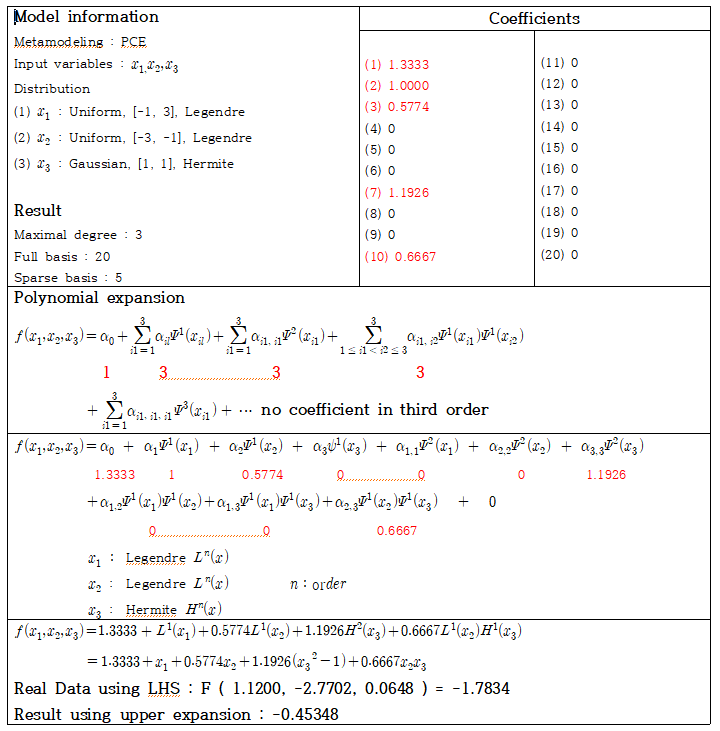# Extract polynomial expansion based on its basis

Hello, UQLabAfter training the PCE Model, I want to obtain polynomial expansion.
Using Legendre and Hermite polynomial, the underlying function was obtained as above.
However, when I calculated it myself using each polynomial basis, it is different from the actual value. Is the expression wrong?

Also, how can I get the basis for the order of Arbitrary PCE?

Additionally, I will do optimization of polynomial function using MATLAB fmincon.
In order to optimization, polynomial formula about underlying function is essential.

I am in the same boat, I also have to apply optimization on the model(predict the new output for each population), I created the model, but can’t save it to predict many outputs for each iteration in optimization.